# International System of Units facts for kids

Kids Encyclopedia Facts
(Redirected from Milli-)

The International System of Units is the standard modern form of the metric system. The name of this system can be shortened or abbreviated to SI, from the French name Système International d'unités.

The International System of Units is a system of measurement based on 7 base units: the metre (length), kilogram (mass), second (time), ampere (electric current), Kelvin (temperature), mole (quantity), and candela (brightness). These base units can be used in combination with each other. This creates SI derived units, which can be used to describe other quantities, such as volume, energy, pressure, and velocity.

The system is used almost globally. Only Myanmar, Liberia, and the United States do not use SI as their official system of measurement. In these countries, though, SI is commonly used in science and medicine.

## History and use

The metric system was created in France after the French Revolution in 1789. The original system only had two standard units, the kilogram and the metre. The metric system became popular amongst scientists.

In the 1860s, James Clerk Maxwell and William Thomson (later known as Lord Kelvin) suggested a system with three base units - length, mass, and time. Other units would be derived from those three base units. Later, this suggestion would be used to create the centimetre-gram-second system of units (CGS), which used the centimetre as the base unit for length, the gram as the base unit for mass, and the second as the base unit for time. It also added the dyne as the base unit for force and the erg as the base unit for energy.

As scientists studied electricity and magnetism, they realized other base units were needed to describe these subjects. By the middle of the 20th century, many different versions of the metric system were being used. This was very confusing.

In 1954, the 9th General Conference on Weights and Measures (CGPM) created the first version of the International System of Units. The six base units that they used were the metre, kilogram, second, ampere, Kelvin, and candela. The seventh base unit, the mole, was added in 1971.

SI is now used almost everywhere in the world, except in the United States, Liberia and Myanmar, where the older imperial units are still widely used. Other countries, most of them historically related to the British Empire, are slowly replacing the old imperial system with the metric system or using both systems at the same time.

## Units of measurement

### Base units

The SI base units are measurements used by scientists and other people around the world. All the other units can be written by combining these seven base units in different ways. These other units are called "derived units".

SI base units
Unit
name
Unit
symbol
Dimension
symbol
Quantity
name
Definition
second s T time
• Prior: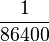$\frac{1}{86400}$ of a day of 24 hours of 60 minutes of 60 seconds
• Interim (1956):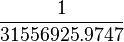$\frac{1}{31556925.9747}$ of the tropical year for 1900 January 0 at 12 hours ephemeris time.
• Current (1967): The duration of 9192631770 periods of the radiation corresponding to the transition between the two hyperfine levels of the ground state of the caesium-133 atom.
metre m L length
• Prior (1793):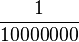$\frac{1}{10000000}$ of the meridian through Paris between the North Pole and the Equator.FG
• Interim (1960): 1650763.73 wavelengths in a vacuum of the radiation corresponding to the transition between the 2p10 and 5d5 quantum levels of the krypton-86 atom.
• Current (1983): The distance travelled by light in vacuum in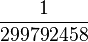$\frac{1}{299792458}$ second.
kilogram
kg M mass
• Prior (1793): The grave was defined as being the mass (then called weight) of one litre of pure water at its freezing point.FG
• Interim (1889): The mass of a small squat cylinder of ~47 cubic centimetres of platinum-iridium alloy kept in the International Bureau of Weights and Measures, Paris, France.
• Current (2019): The kilogram is defined by setting the Planck constant h exactly to 6.62607015×10−34 J⋅s (J = kg⋅m2⋅s−2), given the definitions of the metre and the second. Then the formula would be 1 kg = h6.62607015 × 10-34⋅m2⋅s−1
ampere A I electric current
• Prior (1881): A tenth of the electromagnetic CGS unit of current. The [CGS] electromagnetic unit of current is that current, flowing in an arc 1 cm long of a circle 1 cm in radius, that creates a field of one oersted at the centre. IEC
• Interim (1946): The constant current which, if maintained in two straight parallel conductors of infinite length, of negligible circular cross-section, and placed 1 m apart in vacuum, would produce between these conductors a force equal to 2×10−7 newtons per metre of length.
• Current (2019): The flow of 11.602176634×10−19 times the elementary charge e per second.
kelvin K Θ thermodynamic
temperature
• Prior (1743): The centigrade scale is obtained by assigning 0 °C to the freezing point of water and 100 °C to the boiling point of water.
• Interim (1954): The triple point of water (0.01 °C) defined to be exactly 273.16 K.
• Previous (1967):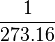$\frac{1}{273.16}$ of the thermodynamic temperature of the triple point of water.
• Current (2019): The kelvin is defined by setting the fixed numerical value of the Boltzmann constant k to 1.380649×10−23 J⋅K−1, (J = kg⋅m2⋅s−2), given the definition of the kilogram, the metre and the second.
mole mol N amount of
substance
• Prior (1900): A stoichiometric quantity which is the equivalent mass in grams of Avogadro's number of molecules of a substance.ICAW
• Interim (1967): The amount of substance of a system which contains as many elementary entities as there are atoms in 0.012 kilogram of carbon-12.
• Current (2019): The amount of substance of exactly 6.02214076×1023 elementary entities. This number is the fixed numerical value of the Avogadro constant, NA, when expressed in the unit mol−1 and is called the Avogadro number.
candela cd J luminous
intensity
• Prior (1946): The value of the new candle (early name for the candela) is such that the brightness of the full radiator at the temperature of solidification of platinum is 60 new candles per square centimetre.
• Current (1979): The luminous intensity, in a given direction, of a source that emits monochromatic radiation of frequency 5.4×1014 hertz and that has a radiant intensity in that direction of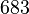${683}$ watt per steradian.
Note: both old and new definitions are approximately the luminous intensity of a whale blubber candle burning modestly bright, in the late 19th century called a "candlepower" or a "candle".
Notes

The Prior definitions of the various base units in the above table were made by the following authorities:

• FG = French Government
• IEC = International Electrotechnical Commission
• ICAW = International Committee on Atomic Weights

All other definitions result from resolutions by either CGPM or the CIPM and are catalogued in the SI Brochure.

### Derived units

Derived units are created by combining the base units. The base units can be divided, multiplied, or raised to powers. Some derived units have special names. Usually these were created to make calculations simpler.

Named units derived from SI base units
Name Symbol Quantity Definition
other units
Definition
SI base units
hertz Hz frequency s−1
newton N force, weight m∙kg∙s−2
pascal Pa pressure, stress N/m2 m−1∙kg∙s−2
joule J energy, work, heat N∙m m2∙kg∙s−2
watt W power, radiant flux J/s m2∙kg∙s−3
coulomb C electric charge s∙A
volt V voltage, electrical potential difference, electromotive force W/A
J/C
m2∙kg∙s−3∙A−1
farad F electrical capacitance C/V m−2∙kg−1∙s4∙A2
ohm Ω electrical resistance, impedance, reactance V/A m2∙kg∙s−3∙A−2
siemens S electrical conductance 1/Ω m−2∙kg−1∙s3∙A2
weber Wb magnetic flux J/A m2∙kg∙s−2∙A−1
tesla T magnetic field strength Wb/m2
V∙s/m2
N/A∙m
kg∙s−2∙A−1
henry H inductance Wb/A
V∙s/A
m2∙kg∙s−2∙A−2
degree Celsius °C temperature relative to 273.15 K TK − 273.15 K
lumen lm luminous flux cd∙sr cd
lux lx illuminance lm/m2 m−2∙cd
becquerel Bq radioactivity (decays per unit time) s−1
gray Gy absorbed dose (of ionizing radiation) J/kg m2∙s−2
sievert Sv equivalent dose (of ionizing radiation) J/kg m2∙s−2
katal kat catalytic activity s−1∙mol

### Prefixes

Very large or very small measurements can be written using prefixes. Prefixes are added to the beginning of the unit to make a new unit. For example, the prefix kilo- means "1000" times the original unit and the prefix milli- means "0.001" times the original unit. So one kilometre is 1000 metres and one milligram is a 1000th of a gram.

Prefix Base 1000 Base 10 Decimal English word Adoption
Name Symbol Short scale Long scale
yotta Y  10008  1024 1000000000000000000000000  septillion  quadrillion 1991
zetta Z  10007  1021 1000000000000000000000  sextillion  trilliard 1991
exa E  10006  1018 1000000000000000000  quintillion  trillion 1975
peta P  10005  1015 1000000000000000  quadrillion  billiard 1975
tera T  10004  1012 1000000000000  trillion  billion 1960
giga G  10003  109 1000000000  billion  milliard 1960
mega M  10002  106 1000000  million 1873
kilo k  10001  103 1000  thousand 1795
hecto h  10002/3  102 100  hundred 1795
deca da  10001/3  101 10  ten 1795
10000  100 1  one
deci d  1000−1/3  10−1 0.1  tenth 1795
centi c  1000−2/3   10−2 0.01  hundredth 1795
milli m  1000−1  10−3 0.001  thousandth 1795
micro μ  1000−2  10−6 0.000001  millionth 1873
nano n  1000−3  10−9 0.000000001  billionth  milliardth 1960
pico p  1000−4  10−12 0.000000000001  trillionth  billionth 1960
femto f  1000−5  10−15 0.000000000000001  quadrillionth  billiardth 1964
atto a  1000−6  10−18 0.000000000000000001  quintillionth  trillionth 1964
zepto z  1000−7  10−21 0.000000000000000000001  sextillionth  trilliardth 1991
yocto y  1000−8  10−24  0.000000000000000000000001  septillionth  quadrillionth 1991

## Images for kidsInternational System of Units Facts for Kids. Kiddle Encyclopedia.